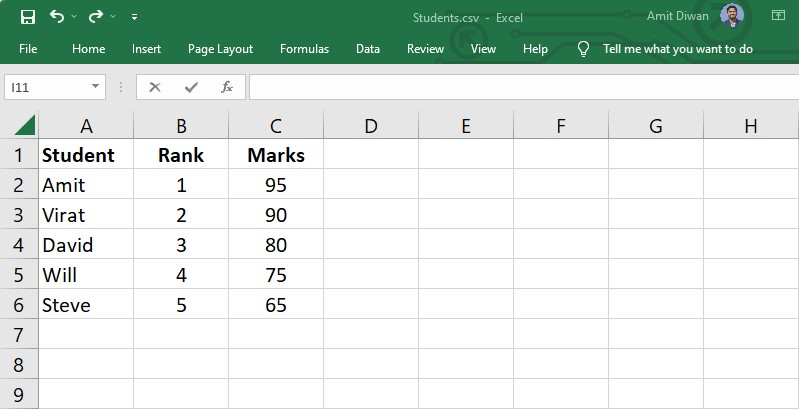## 22 Dec Read CSV in Python Pandas

One of the major benefits of working on Python is the ease of reading and accessing CSV (comma-separated files). For this, Python has the Pandas library. In this lesson, we will learn how to read CSV in Python Pandas using the read_csv() method.

Let’s say we have the following CSV file Students.csv:Let us now see an example to read our CSV file Students.csv using the pandas.read_csv() method in Pandas. First, load the CSV file, read the data, and store it in a Pandas DataFrame:

Output

The above displays the first and the last 5 rows. To display the entire CSV file, use the to_string() method as shown below:

## Display the top n rows of the DataFrame

The head() method will display the first n rows of a DataFrame. The default rows returned are 5.

Let us see an example to display only the top 2 rows. For that, set the parameter of the head() as 2. First, load the CSV file, read the data, and store it in a Pandas DataFrame:

Output

## Display the last n rows of a DataFrame

The tail() method will display the last n rows of a DataFrame. The default rows returned are 5.

Let us see an example to display only the bottom 2 rows. For that, set the parameter of the tail() as 2. First, load the CSV file, read the data, and store it in a Pandas DataFrame:

Output

If you liked the tutorial, spread the word and share the link and our website Studyopedia with others: